## Electric Currents and Magnetic Fields

An electric current will produce a magnetic field, which can be visualized as a series of circular field lines around a wire segment.

### Learning Objectives

Describe shape of a magnetic field produced by an electric current flowing through a wire

### Key Takeaways

#### Key Points

• A wire carrying electric current will produce a magnetic field with closed field lines surrounding the wire.
• Another version of the right hand rules can be used to determine the magnetic field direction from a current—point the thumb in the direction of the current, and the fingers curl in the direction of the magnetic field loops created by it. See.
• The Biot-Savart Law can be used to determine the magnetic field strength from a current segment. For the simple case of an infinite straight current-carrying wire it is reduced to the form $\text{B}=\frac{\mu _{0}\text{I}}{2\pi \text{r}}$.
• A more fundamental law than the Biot-Savart law is Ampere ‘s Law, which relates magnetic field and current in a general way. It is written in integral form as $\oint \text{B}\cdot \text{d}\mathscr{\text{l}}=\mu _{0}\text{I}_{\text{enc}}$, where Ienc is the enclosed current and μ0 is a constant.
• A current-carrying wire feels a force in the presence of an external magnetic field. It is found to be $\text{F}=\text{Bi}\mathscr{\text{l}}\text{sin}\theta$, where ℓ is the length of the wire, i is the current, and θ is the angle between the current direction and the magnetic field.

#### Key Terms

• Biot-Savart Law: An equation that describes the magnetic field generated by an electric current. It relates the magnetic field to the magnitude, direction, length, and proximity of the electric current. The law is valid in the magnetostatic approximation, and is consistent with both Ampère’s circuital law and Gauss’s law for magnetism.
• Ampere’s Law: An equation that relates magnetic fields to electric currents that produce them. Using Ampere’s law, one can determine the magnetic field associated with a given current or current associated with a given magnetic field, providing there is no time changing electric field present.

### Electric Current and Magnetic Fields

Electric current produces a magnetic field. This magnetic field can be visualized as a pattern of circular field lines surrounding a wire. One way to explore the direction of a magnetic field is with a compass, as shown by a long straight current-carrying wire in. Hall probes can determine the magnitude of the field. Another version of the right hand rule emerges from this exploration and is valid for any current segment—point the thumb in the direction of the current, and the fingers curl in the direction of the magnetic field loops created by it.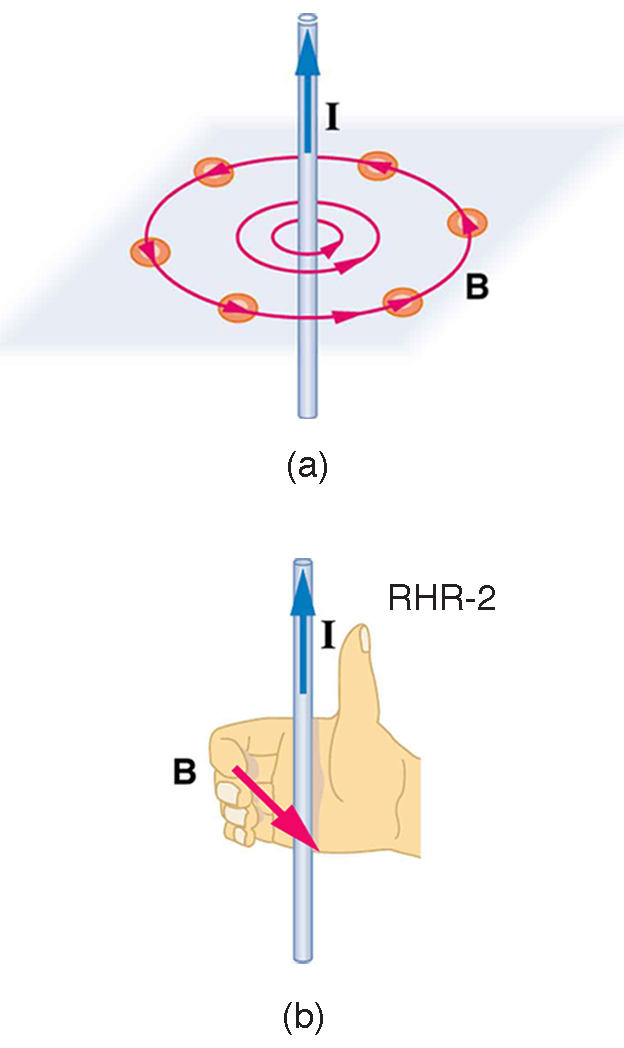Magnetic Field Generated by Current: (a) Compasses placed near a long straight current-carrying wire indicate that field lines form circular loops centered on the wire. (b) Right hand rule 2 states that, if the right hand thumb points in the direction of the current, the fingers curl in the direction of the field. This rule is consistent with the field mapped for the long straight wire and is valid for any current segment.

Magnets and Magnetic Fields: A brief introduction to magnetism for introductory physics students.

### Magnitude of Magnetic Field from Current

The equation for the magnetic field strength (magnitude) produced by a long straight current-carrying wire is:

$\text{B}=\frac{\mu _{0}\text{I}}{2\pi \text{r}}$

For a long straight wire where I is the current, r is the shortest distance to the wire, and the constant 0=4π10−7 T⋅m/A is the permeability of free space. (μ0 is one of the basic constants in nature, related to the speed of light. ) Since the wire is very long, the magnitude of the field depends only on distance from the wire r, not on position along the wire. This is one of the simplest cases to calculate the magnetic field strenght from a current.

The magnetic field of a long straight wire has more implications than one might first suspect. Each segment of current produces a magnetic field like that of a long straight wire, and the total field of any shape current is the vector sum of the fields due to each segment. The formal statement of the direction and magnitude of the field due to each segment is called the Biot-Savart law. Integral calculus is needed to sum the field for an arbitrary shape current. The Biot-Savart law is written in its complete form as:

$\text{B}=\frac{\mu _{\text{o}}\text{I}}{4\pi}\int \frac{\text{d}\mathscr{\text{l}}\times \hat{\text{r}}}{\text{r}^{2}}$

where the integral sums over the wire length where vector dℓ is the direction of the current; r is the distance between the location of dℓ, and the location at which the magnetic field is being calculated; and is a unit vector in the direction of r. The reader may apply the simplifications in calculating the magnetic field from an infinite straight wire as above and see that the Biot-Savart law reduces to the first, simpler equation.

### Ampere’s Law

A more fundamental law than the Biot-Savart law is Ampere’s Law, which relates magnetic field and current in a general way. In SI units, the integral form of the original Ampere’s circuital law is a line integral of the magnetic field around some closed curve C (arbitrary but must be closed). The curve C in turn bounds both a surface S through which the electric current passes through (again arbitrary but not closed—since no three-dimensional volume is enclosed by S), and encloses the current. You can think of the “surface” as the cross-sectional area of a wire carrying current.

The mathematical statement of the law states that the total magnetic field around some path is directly proportional to the current which passes through that enclosed path. It can be written in a number of forms, one of which is given below.

$\oint \text{B}\cdot \text{d}\mathscr{\text{l}}=\mu _{0}\iint_{\text{S}}^{ } \text{J}\cdot \text{dS}=\mu _{0}\text{I}_{\text{enc}}$

where the magnetic field is integrated over a curve (circumfrence of a wire), equivalent to integrating the current density (in amperes per square meter, Am-2) over the cross section area of the wire (which is equal to the permeability constant times the enclosed current Ienc). Ampere’s law is always valid for steady currents and can be used to calculate the B-field for certain highly symmetric situations such as an infinite wire or an infinite solenoid. Ampere’s Law is also a component of Maxwell’s Equations.

### Force on a Current-Carrying Wire

The force on a current carrying wire (as in ) is similar to that of a moving charge as expected since a charge carrying wire is a collection of moving charges. A current-carrying wire feels a force in the presence of a magnetic field. Consider a conductor (wire) of length , cross section A, and charge q which is due to electric current i. If this conductor is placed in a magnetic field of magnitude B which makes an angle with the velocity of charges (current) in the conductor, the force exerted on a single charge q isForce on a Current-Carrying Wire: The right hand rule can be used to determine the direction of the force on a current-carrying wire placed in an external magnetic field.

$\text{F}=\text{qvBsin}\theta$

So, for N charges where

$\text{N}=\text{n}\mathscr{\text{l}}\text{A}$

the force exerted on the conductor is

$\text{f}=\text{FN}=\text{qvBn}\mathscr{\text{l}}\text{Asin}\theta =\text{Bi}\mathscr{\text{l}}\text{sin}\theta$

where i = nqvA. The right hand rule can give you the direction of the force on the wire, as seen in the above figure. Note that the B-field in this case is the external field.

## Permanent Magnets

Permanent magnets are objects made from ferromagnetic material that produce a persistent magnetic field.

### Learning Objectives

Give examples and counterexamples of permanent magnets

### Key Takeaways

#### Key Points

• Permanent magnets are objects made from magnetized material and produce continual magnetic fields. Everyday examples include refrigerator magnets used to hold notes on a refrigerator door.
• Materials that can be magnetized, which are also the ones that are strongly attracted to a magnet, are called ferromagnetic. Examples of these materials include iron, nickel, and cobalt.
• The counterexample to a permanent magnet is an electromagnet, which only becomes magnetized when an electric current flows through it.
• Magnets always have a north pole and a south pole, so if one were to split a permanent magnet in half, two smaller magnets would be created, each with a north pole and south pole.
• Permanent magnets are made from ferromagnetic materials that are exposed to a strong external magnetic field and heated to align their internal microcrystalline structure, making them very hard to demagnetize.

#### Key Terms

• permanent magnet: A material, or piece of such material, which retains its magnetism even when not subjected to any external magnetic fields.
• ferromagnetic: Of a material, such as iron or nickel, that is easily magnetized.
• electromagnet: A magnet which attracts metals only when electrically activated.

### Overview

Recall that a magnet is a material or object that generates a magnetic field. This magnetic field is invisible but is responsible for the most notable property of a magnet: a force that pulls on other ferromagnetic materials, such as iron, and attracts or repels other magnets.

### Types of Magnets

A permanent magnet is an object made from a material that is magnetized and creates its own persistent magnetic field. An everyday example is a refrigerator magnet used to hold notes on a refrigerator door. Materials that can be magnetized, which are also the ones that are strongly attracted to a magnet, are called ferromagnetic. These include iron, nickel, cobalt, some alloys of rare earth metals, and some naturally occurring minerals such as lodestone. Although ferromagnetic materials are the only ones attracted to a magnet strongly enough to be commonly considered magnetic, all other substances respond weakly to a magnetic field, by one of several other types of magnetism. The counterexample to a permanent magnet is an electromagnet, which only becomes magnetized when an electric current flows through it.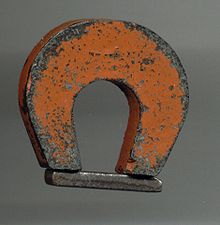Example of a Permanent Magnet: An example of a permanent magnet: a “horseshoe magnet” made of alnico, an iron alloy. The magnet is made in the shape of a horseshoe to bring the two magnetic poles close to each other, to create a strong magnetic field there that can pick up heavy pieces of iron.

### Polarity

All magnets have two poles, one called the north pole and one called the south pole. Like poles repel and unlike polls attract (in analogy to positive and negative charges in electrostatics). North and south poles always exist in pairs (there are no magnetic monopoles in nature), so if one were to split a permanent magnet in half, two smaller magnets would be created, each with a north pole and south pole.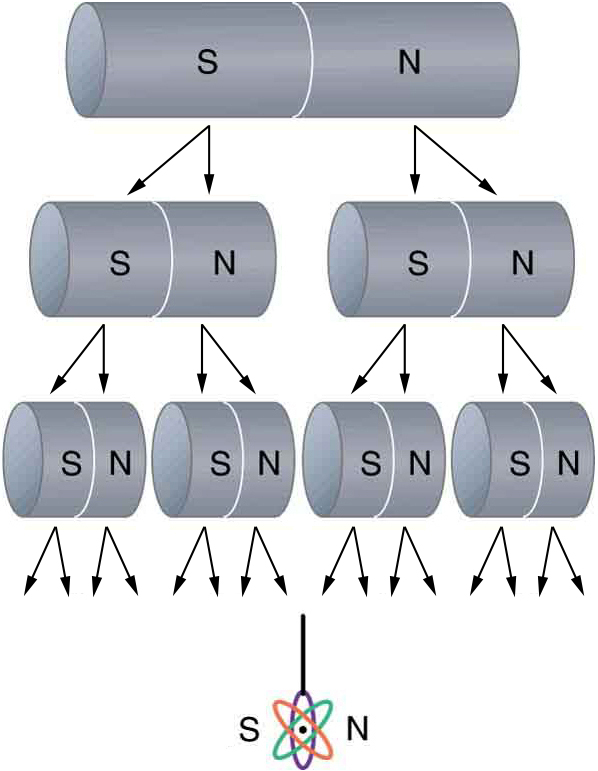North and South Poles Always Come in Pairs: North and south poles always occur in pairs. Attempts to separate them result in more pairs of poles. If we continue to split the magnet, we will eventually get down to an iron atom with a north pole and a south pole—these, too, cannot be separated.

### Manufacturing Permanent Magnets

Ferromagnetic materials can be divided into magnetically “soft” materials like annealed iron, which can be magnetized but do not tend to stay magnetized, and magnetically “hard” materials, which do. Permanent magnets are made from “hard” ferromagnetic materials such as alcino and ferrite that are subjected to special processing in a powerful magnetic field during manufacture, to align their internal microcrystalline structure, making them very hard to demagnetize.

When a magnet is brought near a previously unmagnetized ferromagnetic material, it causes local magnetization of the material with unlike poles closest. (This results in the attraction of the previously unmagnetized material to the magnet. ) On the microscopic scale, there are regions in the unmagnetized ferromagnetic material that act like small bar magnets. In each region the poles of individual atoms are aligned. However, before magnetization these regions are small and randomly oriented throughout the unmagnetized ferromagnetic objects, so there is no net magnetic field. In response to an external magnetic field like the one applied in the above figure, these regions grow and become aligned. This arrangement can become permanent when the ferromagnetic material is heated and then cooled.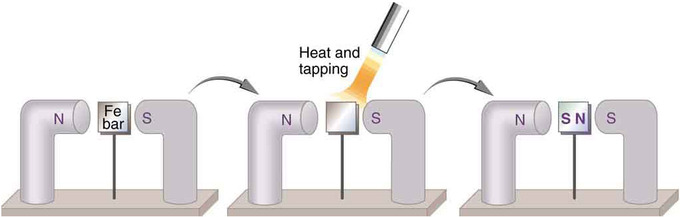Making a Ferromagnet: An unmagnetized piece of iron is placed between two magnets, heated, and then cooled, or simply tapped when cold. The iron becomes a permanent magnet with the poles aligned as shown: its south pole is adjacent to the north pole of the original magnet, and its north pole is adjacent to the south pole of the original magnet. Note that there are attractive forces between the magnets.

## Magnetic Field Lines

Magnetic field lines are useful for visually representing the strength and direction of the magnetic field.

### Learning Objectives

Relate the strength of the magnetic field with the density of the magnetic field lines

### Key Takeaways

#### Key Points

• The magnetic field direction is the same direction a compass needle points, which is tangent to the magnetic field line at any given point.
• The strength of the B-field is inversely proportional to the distance between field lines. It is exactly proportional to the number of lines per unit area perpendicular to the lines.
• A magnetic field line can never cross another field line. The magnetic field is unique at every point in space.
• Magnetic field lines are continuous and unbroken, forming closed loops. Magnetic field lines are defined to begin on the north pole of a magnet and terminate on the south pole.

#### Key Terms

• B-field: A synonym for the magnetic field.
• magnetic field lines: A graphical representation of the magnitude and the direction of a magnetic field.

### Magnetic Field Lines

Einstein is said to have been fascinated by a compass as a child, perhaps musing on how the needle felt a force without direct physical contact. His ability to think deeply and clearly about action at a distance, particularly for gravitational, electric, and magnetic forces, later enabled him to create his revolutionary theory of relativity. Since magnetic forces act at a distance, we define a magnetic field to represent magnetic forces. A pictorial representation of magnetic field lines is very useful in visualizing the strength and direction of the magnetic field. The direction of magnetic field lines is defined to be the direction in which the north end of a compass needle points. The magnetic field is traditionally called the B-field.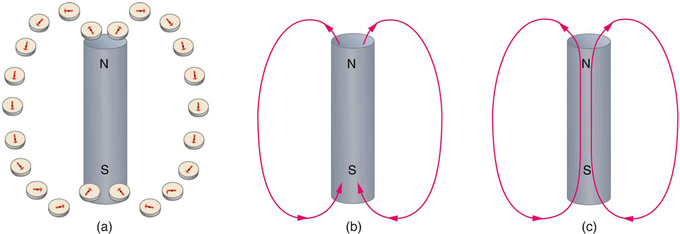Visualizing Magnetic Field Lines: Magnetic field lines are defined to have the direction that a small compass points when placed at a location. (A) If small compasses are used to map the magnetic field around a bar magnet, they will point in the directions shown: away from the north pole of the magnet, toward the south pole of the magnet (recall that Earth’s north magnetic pole is really a south pole in terms of definitions of poles on a bar magnet. ) (B) Connecting the arrows gives continuous magnetic field lines. The strength of the field is proportional to the closeness (or density) of the lines. (C) If the interior of the magnet could be probed, the field lines would be found to form continuous closed loops.

Mapping the magnetic field of an object is simple in principle. First, measure the strength and direction of the magnetic field at a large number of locations (or at every point in space). Then, mark each location with an arrow (called a vector ) pointing in the direction of the local magnetic field with its magnitude proportional to the strength of the magnetic field (producing a vector field). You can “connect” the arrows to form magnetic field lines. The direction of the magnetic field at any point is parallel to the direction of nearby field lines, and the local density of field lines can be made proportional to its strength.

Magnetic field lines are like the contour lines (constant altitude) on a topographic map in that they represent something continuous, and a different mapping scale would show more or fewer lines. An advantage of using magnetic field lines as a representation is that many laws of magnetism (and electromagnetism) can be stated completely and concisely using simple concepts such as the “number” of field lines through a surface. These concepts can be quickly translated to their mathematical form. For example, the number of field lines through a given surface is the surface integral of the magnetic field.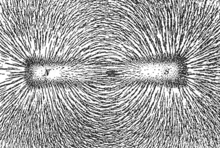Bar Magnet and Magnetic Field Lines: The direction of magnetic field lines represented by the alignment of iron filings sprinkled on paper placed above a bar magnet.

Various phenomena have the effect of “displaying” magnetic field lines as though the field lines are physical phenomena. For example, iron filings placed in a magnetic field line up to form lines that correspond to “field lines. ” Magnetic fields’ lines are also visually displayed in polar auroras, in which plasma particle dipole interactions create visible streaks of light that line up with the local direction of Earth’s magnetic field.

Small compasses used to test a magnetic field will not disturb it. (This is analogous to the way we tested electric fields with a small test charge. In both cases, the fields represent only the object creating them and not the probe testing them. ) Figure 15051 shows how the magnetic field appears for a current loop and a long straight wire, as could be explored with small compasses. A small compass placed in these fields will align itself parallel to the field line at its location, with its north pole pointing in the direction of B. Note the symbols used for field into and out of the paper. We’ll explore the consequences of these various sources of magnetic fields in further sections.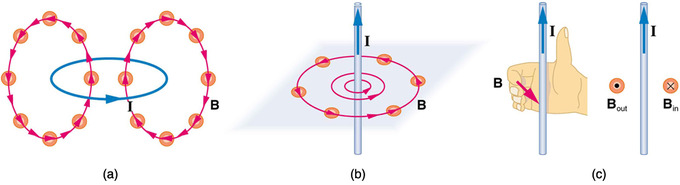Mapping Magnetic Field Lines: Small compasses could be used to map the fields shown here. (A) The magnetic field of a circular current loop is similar to that of a bar magnet. (B) A long and straight wire creates a field with magnetic field lines forming circular loops. (C) When the wire is in the plane of the paper, the field is perpendicular to the paper. Note that the symbols used for the field pointing inward (like the tail of an arrow) and the field pointing outward (like the tip of an arrow).

Extensive exploration of magnetic fields has revealed a number of hard-and-fast rules. We use magnetic field lines to represent the field (the lines are a pictorial tool, not a physical entity in and of themselves). The properties of magnetic field lines can be summarized by these rules:

1. The direction of the magnetic field is tangent to the field line at any point in space. A small compass will point in the direction of the field line.
2. The strength of the field is proportional to the closeness of the lines. It is exactly proportional to the number of lines per unit area perpendicular to the lines (called the areal density).
3. Magnetic field lines can never cross, meaning that the field is unique at any point in space.
4. Magnetic field lines are continuous, forming closed loops without beginning or end. They go from the north pole to the south pole.

The last property is related to the fact that the north and south poles cannot be separated. It is a distinct difference from electric field lines, which begin and end on the positive and negative charges. If magnetic monopoles existed, then magnetic field lines would begin and end on them.

## Geomagnetism

Earth’s magnetic field is caused by electric currents in the molten outer core and varies with time.

### Learning Objectives

Explain the origin of the Earth’s magnetic field and its importance for the life on Earth

### Key Takeaways

#### Key Points

• Earth is largely protected from the solar wind, a stream of energetic charged particles emanating from the sun, by its magnetic field. These particles would strip away the ozone layer, which protects Earth from harmful ultraviolet rays.
• Earth’s magnetic field is generated by a feedback loop in the liquid outer core: Current loops generate magnetic fields; a changing magnetic field generates an electric field; and the electric and magnetic fields exert a force on the charges that are flowing in currents (the Lorentz force).
• The geomagnetic field varies with time. Currents in the ionosphere and magnetosphere cause changes over short time scales, while dramatic geomagnetic reversald (where the North and South poles switch locations) occur at apparently random intervals ranging from 0.1 to 50 million years.

#### Key Terms

• dynamo: A mechanism by which a celestial body such as Earth or a star generates a magnetic field over astronomical timescales via a rotating, convecting, and electrically conducting fluid.

### The Structure of Earth’s Magnetic Field

Earth is largely protected from the solar wind, a stream of energetic charged particles emanating from the sun, by its magnetic field, which deflects most of the charged particles. These particles would strip away the ozone layer, which protects Earth from harmful ultraviolet rays. The region above the ionosphere, and extending several tens of thousands of kilometers into space, is called the magnetosphere. This region protects Earth from cosmic rays that would strip away the upper atmosphere, including the ozone layer that protects our planet from harmful ultraviolet radiation. The magnetic field strength ranges from approximately 25 to 65 microteslas (0.25 to 0.65 G; by comparison, a strong refrigerator magnet has a field of about 100 G). The intensity of the field is greatest near the poles and weaker near the equator. An isodynamic chart of Earth’s magnetic field, shows a minimum intensity over South America while there are maxima over northern Canada, Siberia, and the coast of Antarctica south of Australia. Near the surface of Earth, its magnetic field can be closely approximated by the field of a magnetic dipole positioned at the center of Earth and tilted at an angle of about 10° with respect to the rotational axis of Earth.

### Physical Origin

Earth’s magnetic field is mostly caused by electric currents in the liquid outer core, which is composed of highly conductive molten iron. A magnetic field is generated by a feedback loop: Current loops generate magnetic fields (Ampère’s law); a changing magnetic field generates an electric field (Faraday’s law); and the electric and magnetic fields exert a force on the charges that are flowing in currents (the Lorentz force). These effects can be combined into a partial differential equation called the magnetic induction equation:

$\frac{\partial \mathbf{\text{B}}}{\partial \text{t}} = \eta \mathbf{\nabla}^2 \mathbf{\text{B}} + \mathbf{\nabla}\times (\mathbf{\text{u}} \times \mathbf{\text{B}})$

In this equation u is the velocity of the fluid, B is the magnetic field, and eta is the magnetic diffusivity. The first term on the right hand side of the induction equation is a diffusion term. If Earth’s dynamo shut off, the dipole part would disappear in a few tens of thousands of years. The motion of the molten outer iron core is sustained by convection, or motion driven by buoyancy. The temperature increases toward the center of Earth, and the higher temperature of the fluid lower down makes it buoyant. The Coriolis effect, caused by the overall planetary rotation, tends to organize the flow into rolls aligned along the north-south polar axis.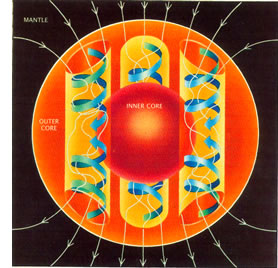Origin of Earth’s Magnetic Field: A schematic illustrating the relationship between motion of conducting fluid, organized into rolls by the Coriolis force, and the magnetic field the motion generates.

Electric currents induced in the ionosphere generate magnetic fields (ionospheric dynamo region). Such a field is always generated near where the atmosphere is closest to the sun, causing daily alterations that can deflect surface magnetic fields by as much as one degree. Typical daily variations of field strength are about 25 nanoteslas (nT), with variations over a few seconds of typically around 1 nT.

### Time Variations

The geomagnetic field changes on time scales from milliseconds to millions of years. Shorter time scales mostly arise from currents in the ionosphere (ionospheric dynamo region) and magnetosphere, and some changes can be traced to geomagnetic storms or daily variations in currents. Changes over time scales of a year or more mostly reflect changes in Earth’s interior, particularly the iron-rich core. Frequently, Earth’s magnetosphere is hit by solar flares causing geomagnetic storms, provoking displays of aurorae. At present, the overall geomagnetic field is becoming weaker; the present strong deterioration corresponds to a 10 to 15 percent decline over the last 150 years and has accelerated in the past several years. Geomagnetic intensity has declined almost continuously from a maximum 35 percent above the modern value achieved approximately 2,000 years ago. Earth’s magnetic North Pole is drifting from northern Canada toward Siberia with a presently accelerating rate—10 km per year at the beginning of the 20th century, up to 40 km per year in 2003, and since then has only accelerated.

Although Earth’s field is generally well approximated by a magnetic dipole with its axis near the rotational axis, there are occasional dramatic events where the North and South geomagnetic poles trade places. These events are called geomagnetic reversals. Evidence for these events can be found worldwide in basalts, sediment cores taken from the ocean floors, and seafloor magnetic anomalies. Reversals occur at apparently random intervals ranging from less than 0.1 million years to as much as 50 million years. The most recent such event, called the Brunhes-Matuyama reversal, occurred about 780,000 years ago.